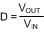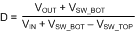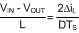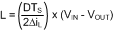SNVS579L February   2009  – May 2018

PRODUCTION DATA.

1. Features
2. Applications
3. Description
1.     Device Images
4. Revision History
5. Pin Configuration and Functions
6. Specifications
7. Detailed Description
1. 7.1 Overview
2. 7.2 Functional Block Diagram
3. 7.3 Feature Description
4. 7.4 Device Functional Modes
8. Application and Implementation
1. 8.1 Application Information
2. 8.2 Typical Applications
1. 8.2.1 LM26420X 2.2-MHz, 0.8-V Typical High-Efficiency Application Circuit
2. 8.2.2 LM26420X 2.2-MHz, 1.8-V Typical High-Efficiency Application Circuit
3. 8.2.3 LM26420X 2.2-MHz, 2.5-V Typical High-Efficiency Application Circuit
4. 8.2.4 LM26420Y 550 kHz, 0.8-V Typical High-Efficiency Application Circuit
5. 8.2.5 LM26420Y 550-kHz, 1.8-V Typical High-Efficiency Application Circuit
6. 8.2.6 LM26420Y 550-kHz, 2.5-V Typical High-Efficiency Application Circuit
9. Power Supply Recommendations
10. 10Layout
11. 11Device and Documentation Support
12. 12Mechanical, Packaging, and Orderable Information

#### 8.2.1.2.2 Inductor Selection

The duty cycle (D) can be approximated as the ratio of output voltage (VOUT) to input voltage (VIN):

Equation 5.The voltage drop across the internal NMOS (SW_BOT) and PMOS (SW_TOP) must be included to calculate a more accurate duty cycle. Calculate D by using the following formulas:

Equation 6.VSW_TOP and VSW_BOT can be approximated by:

Equation 7. VSW_TOP = IOUT × RDSON_TOP
Equation 8. VSW_BOT = IOUT × RDSON_BOT

The inductor value determines the output ripple voltage. Smaller inductor values decrease the size of the inductor, but increase the output ripple voltage. An increase in the inductor value decreases the output ripple current.

One must ensure that the minimum current limit (2.4 A) is not exceeded, so the peak current in the inductor must be calculated. The peak current (ILPK) in the inductor is calculated by:

Equation 9. ILPK = IOUT + ΔiLFigure 36. Inductor Current
Equation 10.In general,

Equation 11. ΔiL = 0.1 × (IOUT) → 0.2 × (IOUT)

If ΔiL = 20% of 2 A, the peak current in the inductor is 2.4 A. The minimum ensured current limit over all operating conditions is 2.4 A. One can either reduce ΔiL, or make the engineering judgment that zero margin is safe enough. The typical current limit is 3.3 A.

The LM26420 operates at frequencies allowing the use of ceramic output capacitors without compromising transient response. Ceramic capacitors allow higher inductor ripple without significantly increasing output ripple voltage. See Output Capacitor section for more details on calculating output voltage ripple. Now that the ripple current is determined, the inductance is calculated by:

Equation 12.Where

Equation 13.When selecting an inductor, make sure that it is capable of supporting the peak output current without saturating. Inductor saturation results in a sudden reduction in inductance and prevent the regulator from operating correctly. The peak current of the inductor is used to specify the maximum output current of the inductor and saturation is not a concern due to the exceptionally small delay of the internal current limit signal. Ferrite based inductors are preferred to minimize core losses when operating with the frequencies used by the LM26420. This presents little restriction because the variety of ferrite-based inductors is huge. Lastly, inductors with lower series resistance (RDCR) provides better operating efficiency. For recommended inductors see Table 2.